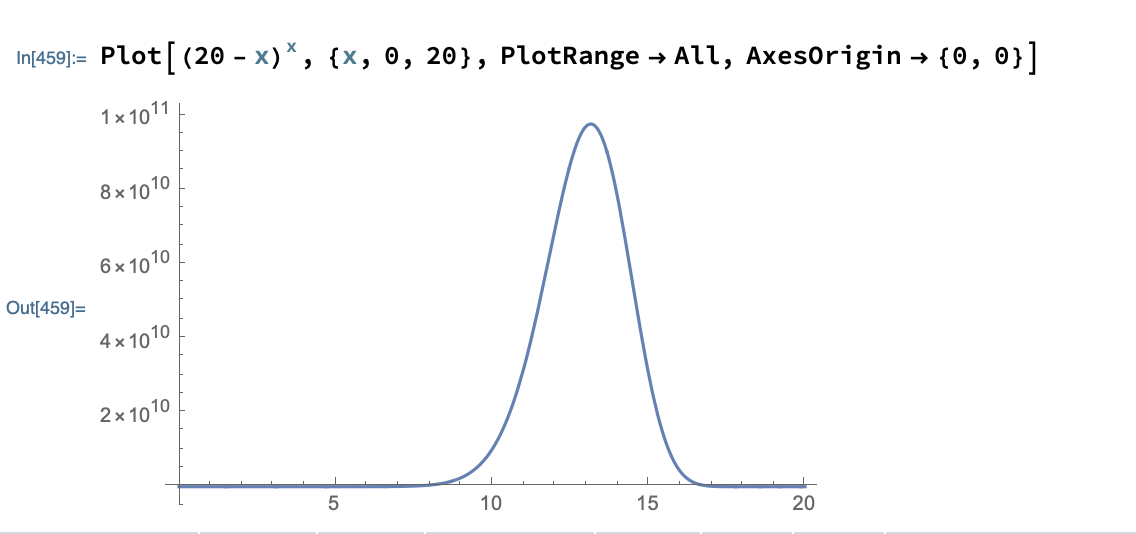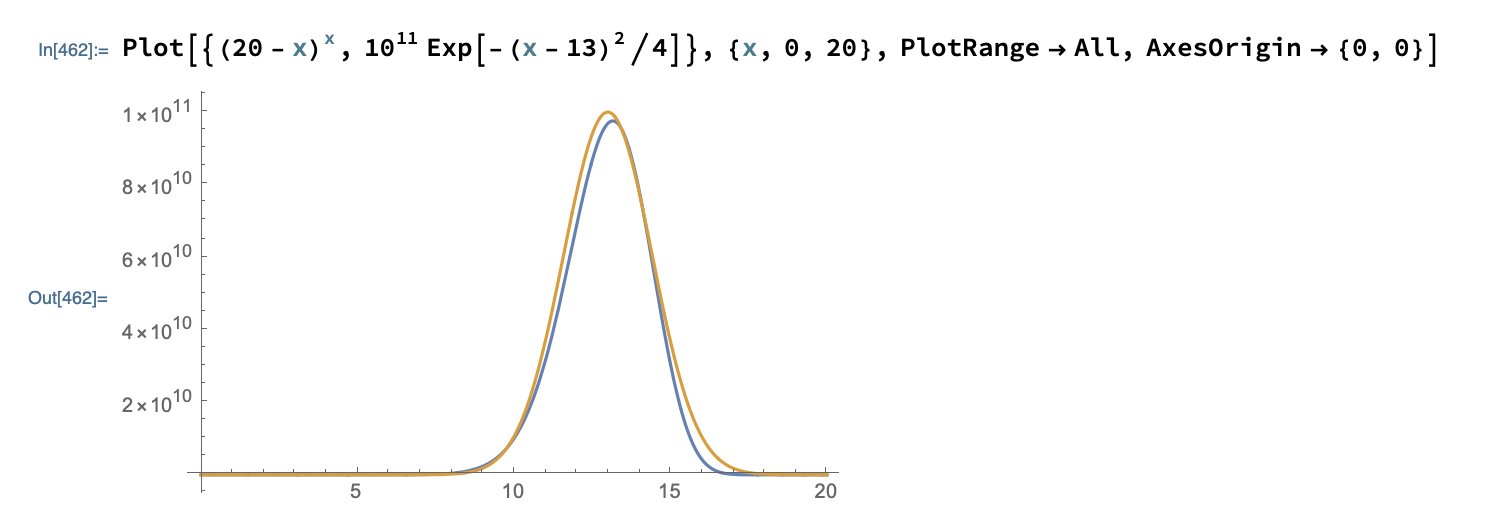# Bounds of $1^n + 2^{n-1} + 3^{n-2} + \cdots + n^1$

I want to estimate the value of this sequence for large $$n$$ (with a reasonable lower bound and upper bound).

That is, can we find a function $$f(n)$$ such that $$\frac{1^n + 2^{n-1} + 3^{n-2} + \cdots + n^1}{f(n)} \rightarrow 1?$$

I tried to approximate $$(n - x)^x$$ as a Gaussian, look at how close it is visually, but the manipulation escapes me.

Here is the case where $$n=20$$:Addendum: This post might be relevant.

• The largest term is at $x$ where $x+x\ln x=n$. The second derivative will let you find the standard deviation. Jul 14 '20 at 6:38
• Try Laplace's method: the sum $\sum_{j=1}^n j^{n-j+1}$ can be approximated by $$\int_1^n x^{n-x+1} dx \approx \int_1^n e^{(n-x+1) \operatorname{ln}(x)}dx.$$ en.wikipedia.org/wiki/Laplace%27s_method Jul 14 '20 at 6:40
• If $$F(x)=e^x+2\left(\dfrac{e^{x}}{2}\right)^2+3\left(\dfrac{e^{x}}{3}\right)^3+\cdots+n\left(\dfrac{e^{x}}{n}\right)^n,$$ then $$F^{(n)}(0)=1^n + 2^{n-1} + 3^{n-2} + \cdots + (n-1)^2+n.$$ Jul 14 '20 at 7:37
• With the optimized parameters, the plot is much more symmetric even if, compared to your approximation, the infinite norm is only divided by a factor of 2. Jul 15 '20 at 15:17
• – Sil
Jul 16 '20 at 17:48

If you let $$m_n>1$$ be the unique solution to $$m_n = \frac{n}{1+\ln(m_n)}$$ and $$S_n=\sum_{k=1}^n k^{n-k}$$ (I have shifted n by one as in your plots), then $$\lim_{n\rightarrow +\infty} \frac{1}{S_n} m_n^{n-m_n+1} \sqrt{\frac{2\pi}{m_n+n}} =1.$$ This is what you would suspect using the method of Laplace, writing $$x^{n-x}=e^{f_n(x)}$$ approximating $$f_n$$ by a parabola at the maximum (which is for $$x=m_n$$) and calculating the resulting gauss integral. I didn't check rigorously if the third order correction to the Laplace formula is negligible but numerically the above seems to hold.

EDIT: Let me outline a sketch of a proof (filling in details would probably amount to writing about 3 pages, dense with formulae). It follows more or less known lines in the case of Stirlings formula.

Step 1: Show that $$\sum_{k=1}^n k^{n-k}$$ is equivalent to the integral $$\int_1^n e^{f_n(x)}dx$$ with $$f_n(x)=(n-x)\ln(x)$$ as $$n\rightarrow +\infty$$. This is not so difficult.

Step 2: We write $$m=m_n$$. Substituting $$x=m+ t \frac{m}{\sqrt{m+n}}$$ and using $$\ln m = \frac{n-m}{m}$$ one obtains after some algebra: \begin{align} f_n(x) & = m \left( \frac{n-m}{m} - \frac{t}{\sqrt{n+m}}\right) \left( \frac{n-m}{m} + \ln \left(1+ \frac{t}{\sqrt{n+m}} \right)\right)\\ & = m\left(\frac{n-m}{m}\right)^2 -\frac{t^2}{2} + O\left(\frac{t^3}{\sqrt{n}}\right)\end{align} and then $$\int_1^n e^{f_n(x)} dx = \frac{m_n^{n-m_n+1}}{\sqrt{n+m}} \int_{-l_n}^{u_n} \exp \left( -\frac{t^2}{2} + O \left( \frac{t^3}{\sqrt{n}}\right)\right)\; dt.$$ Here, $$l_n,u_n\rightarrow +\infty$$ as $$n$$ goes to infinity and pointwise the integrand goes to $$e^{-t^2/2}$$.

Step 3: Show that the integrand is bounded uniformly in $$n$$ by an integrable function and apply Lebesgue dominated convergence to conclude the proof.

For Step 3, note that a Gaussian function would not work as a dominating function due to the logarithmic tail in $$f_n$$. You need to construct the dominating function in a more clever way. This is where calculations become more ugly and I leave it aside.

• (+1) It is a nice solution. It should be $m_n + n$ rather than $m+n$? Also, $m_n = \mathrm{e}^{W(n\mathrm{e}) - 1} = \frac{n}{W(n\mathrm{e})}$ where $W$ is the Lambert W function. Hope to see rigorous proofs of the limit equal to one. Jul 19 '20 at 2:53
• @RiverLi Thanks. Ii is indeed $m_n$. I have corrected. The problem is nice, but looks to me more complicated to prove than Stirling's formula (more terms to control) and Stirling's itself requires a couple of pages to prove rigorously. Jul 19 '20 at 7:00
• @RiverLi In case you are still interested. I have added an outline of a proof. Jul 20 '20 at 6:49
• It is nice. Thanks. Jul 20 '20 at 8:07

Partial answer: $$(a-x)^x= Ae^{-(x-b)^2/c}+O(|x-b|^3)$$

Taking logs $$x\ln(a-x)=\ln A-(x-b)^2/c$$

Let $$x=b+y$$, then expanding out gives $$b\ln(a-b)+y\ln(a-b)-\frac{b}{a-b}y-\frac{1}{a-b}y^2-\frac{b}{2(a-b)^2}y^2+O(y^3)=\ln A-\frac{y^2}{c}$$

Comparing the terms we need $$\ln A=b\ln(a-b),$$ $$(a-b)\ln(a-b)=b,$$ $$\frac{1}{c}=\frac{1}{a-b}+\frac{b}{2(a-b)^2}=\frac{2a-b}{2(a-b)^2}.$$ These determine $$b,A,c$$ for the best fit.

Checking with the example $$a=20$$, gives $$b=13.16$$, $$c=-3.49$$, $$A=9.7\times10^{10}$$.

This suggests we take $$A\sqrt{\pi c}=\sqrt{2\pi}\frac{(a-b)^{b+1}}{\sqrt{2a-b}}$$ as the approximation of the sum.

Comparing the sum for $$n=a=10,\ldots,20$$ gives

$$Sum(n)=11377, 49863, 232768, 1151914, 6018785, 33087205, 190780212, 1150653920, 7241710929, 47454745803, 323154696184$$

$$Approx(n)=11374, 49845, 232672, 1151410, 6016080, 33072000, 190692000, 1150120000, 7238320000, 47432600000, 323004000000$$

• H.H.Rugh's answer is the same, with $m_n=a-b$. Jul 14 '20 at 9:09
• I used your answer to make all analytical. Cheers :-) Jul 15 '20 at 9:51

Starting from @Chrystomath's answer, for a given value of $$a$$ we have analyical expressions for $$A$$, $$b$$ and $$c$$.

The trick is to rewrite his/her second equation $$(a-b)\ln(a-b)=b$$ $$(a-b)+(a-b)\ln(a-b)=a \implies k+k \log(k)=a \implies k=\frac{a}{W(e a)}$$ where $$W(.)$$ is Lambert function. So, this gives $$\color{blue}{A=k^{a-k}\qquad b=a-k \qquad c=\frac{2 k^2}{a+k}}\qquad \text{with} \qquad \color{red}{k=\frac{a}{W(e a)}}$$ and then $$A\sqrt{\pi c}= k^{a-k+1} \sqrt{\frac{2 \pi}{a+k}}$$ Rounding the numbers, for $$a=20$$, this generates the sequence $$\{2,4,9,23,66,210,733,2780,11374,49845,232672,1151412,6016082,33072048,190691716, 1150116697,7238323772,47432585137,323004401255,2281724622065, 16693240814087\}$$ instead of $$\{1,3,8,22,65,209,732,2780,11377,49863,232768,1151914,6018785,33087205,190780212,11 50653920,7241710929,47454745803,323154696184, 2282779990494, 16700904488705\}$$

Edit

All the work can be done without expansions but instead function identification at a single point.

Consider $$f(x)=(a-x)^x$$ $$f'(x)=(a-x)^x \left(\log (a-x)-\frac{x}{a-x}\right)$$ $$f''(x)=(a-x)^{x-2} \left((a-x) \log (a-x) ((a-x) \log (a-x)-2 x)-2 a+x^2+x\right)$$ $$g(x)=A e^{-\frac{(x-b)^2}{c}}$$ $$g'(x)=-\frac{2 A (x-b) e^{-\frac{(x-b)^2}{c}}}{c}$$ $$g''(x)=-\frac{2 A e^{-\frac{(b-x)^2}{c}} \left(c-2 (b-x)^2\right)}{c^2}$$ Compute $$x_*$$ corresponding to $$f'(x)=0$$ and then solve for $$(A,b,c)$$ the equations $$f(x_*)=g(x_*) \qquad \qquad f'(x_*)=g'(x_*) \qquad \qquad f''(x_*)=g''(x_*)$$ For sure, this leads to the same results.